How Cheenta works to ensure student success?
Explore the Back-Story

# Directional Derivative : IIT JAM 2018 Problem 42# Understand the problem

[/et_pb_text][et_pb_text _builder_version="4.1" text_font="Raleway||||||||" background_color="#f4f4f4" custom_margin="10px||10px" custom_padding="10px|20px|10px|20px" box_shadow_style="preset2"]Let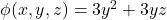for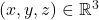Then the absolute value of the directional derivative ofin the direction of the line, at the point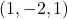is _____

[/et_pb_code][et_pb_accordion open_toggle_text_color="#0c71c3" _builder_version="4.1" toggle_font="||||||||" body_font="Raleway||||||||" text_orientation="center" custom_margin="10px||10px"][et_pb_accordion_item title="Source of the problem" open="on" _builder_version="4.1"]IIT JAM 2018[/et_pb_accordion_item][et_pb_accordion_item title="Topic" _builder_version="4.1" open="off"]Directional Derivative [/et_pb_accordion_item][et_pb_accordion_item title="Difficulty Level" _builder_version="4.1" open="off"]Easy[/et_pb_accordion_item][et_pb_accordion_item title="Suggested Book" _builder_version="4.1" open="off"]

Generalized Directional Derivatives  By Pastor Karel

[/et_pb_text][et_pb_tabs active_tab_background_color="#0c71c3" inactive_tab_background_color="#000000" _builder_version="4.1" tab_text_color="#ffffff" tab_font="||||||||" background_color="#ffffff" hover_enabled="0"][et_pb_tab title="Hint 0" _builder_version="3.22.4"]Do you really need a hint? Try it first!

[/et_pb_tab][et_pb_tab title="Hint 1" _builder_version="4.1" hover_enabled="0"] The rate of change of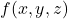in the direction of the unit vector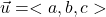is called the directional derivative and is denoted by. The defination of directional derivative is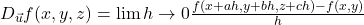. Here if you consider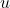to beand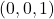then we will getto be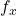,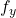and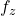respectively. If you observe closely, this is nothing but differentiating the function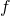w.r.t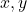and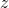keeping all the other thing constant.

Now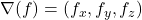by the above definition can you try to solve the problem ???????

[/et_pb_tab][et_pb_tab title="Hint 2" _builder_version="4.1"]

The points on the straight line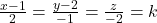So any point on the straight line would be of the form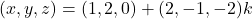Here the direction of the straight line would be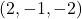Now we have to consider the unit vector in that direction and so the unit vector would beHere the absolute value of the directional derivative would be. Would you like to calculate this dot product?

[/et_pb_tab][et_pb_tab title="Hint 3" _builder_version="4.1"]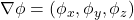i.e the co-ordinates involving partial derivative of. So,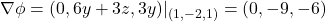Then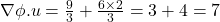(Ans) .

# Similar Problems

[/et_pb_text][et_pb_post_slider include_categories="12" image_placement="left" _builder_version="4.1"][/et_pb_post_slider][et_pb_divider _builder_version="3.22.4" background_color="#0c71c3"][/et_pb_divider][/et_pb_column][/et_pb_row][/et_pb_section]

# Understand the problem

[/et_pb_text][et_pb_text _builder_version="4.1" text_font="Raleway||||||||" background_color="#f4f4f4" custom_margin="10px||10px" custom_padding="10px|20px|10px|20px" box_shadow_style="preset2"]LetforThen the absolute value of the directional derivative ofin the direction of the line, at the pointis _____

[/et_pb_code][et_pb_accordion open_toggle_text_color="#0c71c3" _builder_version="4.1" toggle_font="||||||||" body_font="Raleway||||||||" text_orientation="center" custom_margin="10px||10px"][et_pb_accordion_item title="Source of the problem" open="on" _builder_version="4.1"]IIT JAM 2018[/et_pb_accordion_item][et_pb_accordion_item title="Topic" _builder_version="4.1" open="off"]Directional Derivative [/et_pb_accordion_item][et_pb_accordion_item title="Difficulty Level" _builder_version="4.1" open="off"]Easy[/et_pb_accordion_item][et_pb_accordion_item title="Suggested Book" _builder_version="4.1" open="off"]

Generalized Directional Derivatives  By Pastor Karel

[/et_pb_text][et_pb_tabs active_tab_background_color="#0c71c3" inactive_tab_background_color="#000000" _builder_version="4.1" tab_text_color="#ffffff" tab_font="||||||||" background_color="#ffffff" hover_enabled="0"][et_pb_tab title="Hint 0" _builder_version="3.22.4"]Do you really need a hint? Try it first!

[/et_pb_tab][et_pb_tab title="Hint 1" _builder_version="4.1" hover_enabled="0"] The rate of change ofin the direction of the unit vectoris called the directional derivative and is denoted by. The defination of directional derivative is. Here if you considerto beandthen we will getto be,andrespectively. If you observe closely, this is nothing but differentiating the functionw.r.tandkeeping all the other thing constant.

Nowby the above definition can you try to solve the problem ???????

[/et_pb_tab][et_pb_tab title="Hint 2" _builder_version="4.1"]

The points on the straight lineSo any point on the straight line would be of the formHere the direction of the straight line would beNow we have to consider the unit vector in that direction and so the unit vector would beHere the absolute value of the directional derivative would be. Would you like to calculate this dot product?

[/et_pb_tab][et_pb_tab title="Hint 3" _builder_version="4.1"]i.e the co-ordinates involving partial derivative of. So,Then(Ans) .

# Similar Problems

[/et_pb_text][et_pb_post_slider include_categories="12" image_placement="left" _builder_version="4.1"][/et_pb_post_slider][et_pb_divider _builder_version="3.22.4" background_color="#0c71c3"][/et_pb_divider][/et_pb_column][/et_pb_row][/et_pb_section]

This site uses Akismet to reduce spam. Learn how your comment data is processed.

### Knowledge Partner